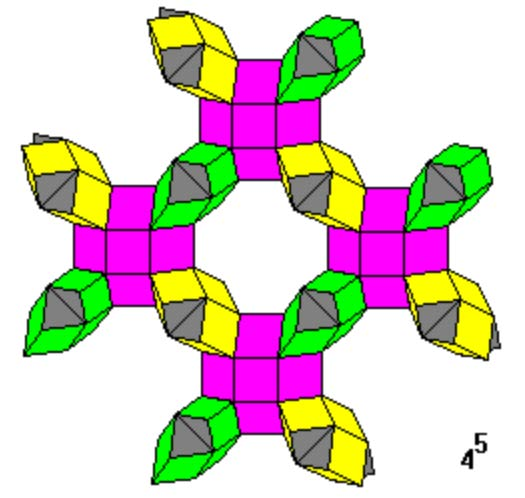Acronym ... Name Wells' "hyperbolic" {4,5} polyhedron© Vertex figure  (non-regular, non-flat) Dihedral angles (at margins) at base edges:   arccos(-sqrt(2/3)) = 144.735610° at equatorial edges:   arccos(-1/3) = 109.471221° at lacing edges:   ...

Despite of being uniform and additionally having a single face shape only, these fall into different classes of symmetry.

This uniform skew polyhedron looks like being obtained from all the squares of a structure, built of interlocking squobcues. None the less those aren't true according Johnson solids, rather those are variants thereof. In fact the axial height h of those bicupolae has to be chosen such that it matches to the equatorial triangle-triangle edges c, while all squares remain regular. This then amounts in values c = h = 2/sqrt(3) = 1.154701 > 1

Incidence matrix

(N → ∞)

4N |  2  2  1 | 1  4
---+----------+-----
2 | 4N  *  * | 1  1  base edges
2 |  * 4N  * | 0  2  lacing edges
2 |  *  * 2N | 0  2  equatorial edges
---+----------+-----
4 |  4  0  0 | N  *  base squares of orthobicupolae
4 |  1  2  1 | * 4N  lacing squares of orthobicupolae